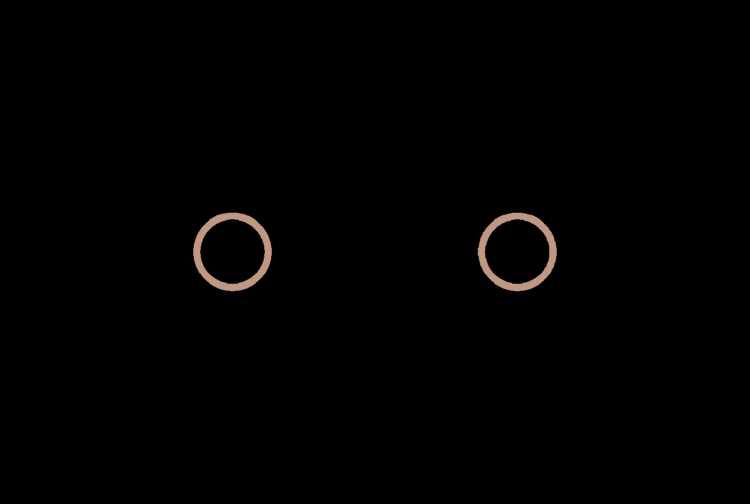# Binary Black Hole Inspiral

Two black holes in a mutually orbiting binary system both have mass $M$ and orbit at radius $R$ to their center of rotation.What is the magnitude of the rate of change of their radius (the rate at which they inspiral due to energy loss to gravitational radiation)?

Assumptions and Details:

• The power radiated in gravitational waves by an inspiraling binary is $P = -\dfrac25 \dfrac{G^4 M^5}{c^5 R^5}.$
• Apart from the expression for gravitational wave radiation, assume Newtonian mechanics applies.
×